## Thursday 22 March 2018

### Three Cones and a Sphere

Three identical cones with radius R_cone and height h are placed such that the circular bases touch one another (i.e. are mutually tangent). A sphere is then placed in the space between the cones. The sphere is touching the ground and all three cones, and the top of the sphere is at the same height as the vertices of the cones. What is the relation between h, R_cone, and R_sphereA sphere resting on three identical cones with mutually tangent bases.

For the sphere to touch the ground and also match the height of cones, it's diameter must equal h.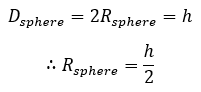Condition 1 for a sphere in contact with the ground and the three cones, and with its top at the same height as the vertices.

Next, we know that the side of the cone is also a point of tangency on the sphere. The vertical section at the contact point will look like this: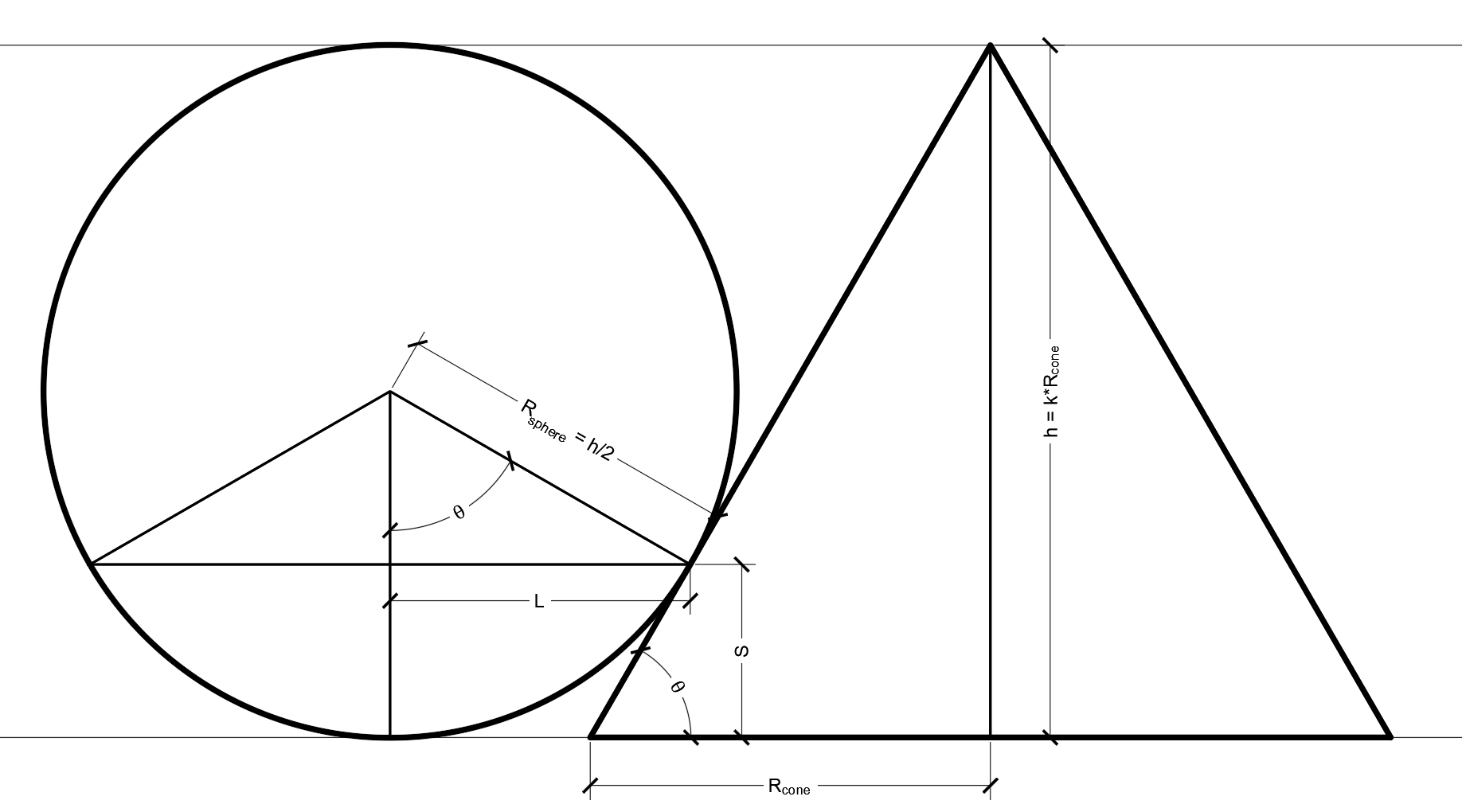Cross-section through the sphere and one cone at the contact point.

The radius of the sphere has already been defined. We also relate the height of the cone to its radius by the factor k = h / R_cone. That way k = tan[θ].

The sagitta S is equal to: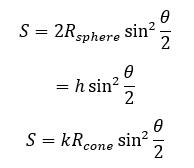Expression for the sagitta (vertical distance from the bottom of the sphere to the point of contact with the cone)

The half-chord L is the radius of the circle through the three contact points between the sphere and cones. At the ground level there's a small space between the three cones. The largest circle that can fit inside that space is sometimes called an "inner Soddy circle" and its radius can be determined from Descartes' theorem. We'll call this L_0.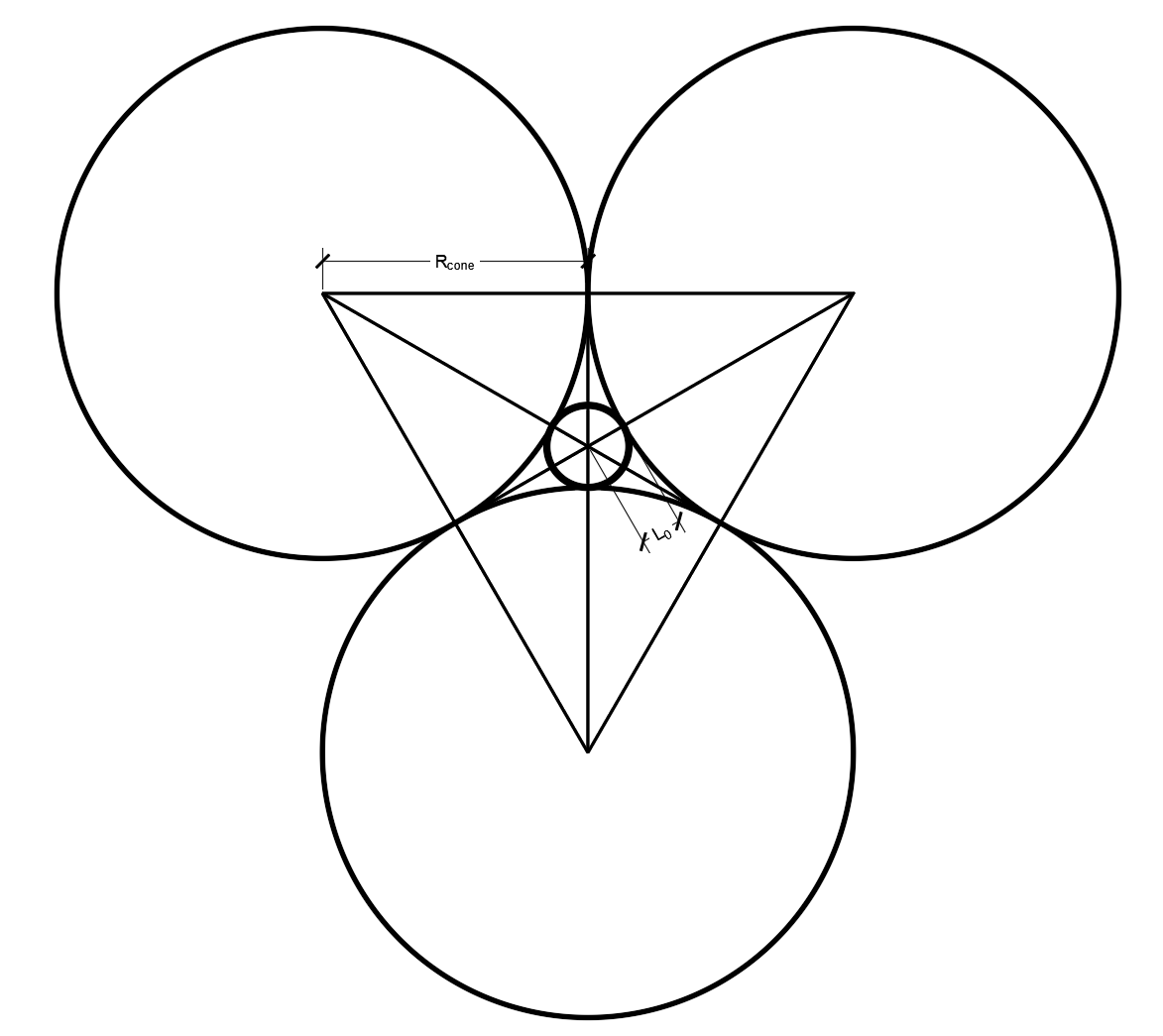View of the three mutually tangent circular bases and the inner Soddy circle between them.

From Descartes' theorem we get: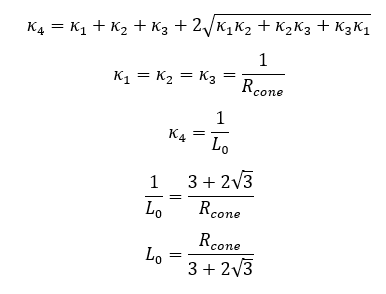Radius of the inner Soddy circle at the ground level.

The radius of the horizontal circle touching all three cones increases with height z above the base of the cone, varying linearly. The amount it increases is equal to the decrease in the cross-sectional radius of the cone at the same height, relative to the radius at the base. Therefore: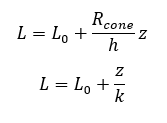Expression for the radius of the circle just touching all three cones at height z above the base.

But in this special case z must be equal to the sagitta S, thus: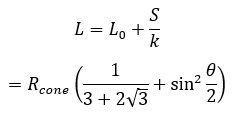Equation 1

From trigonometry we also get: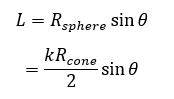Equation 2

Solving for θ such that Equation 1 = Equation 2: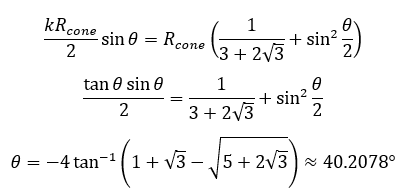Condition 2 for a sphere in contact with ground and the three cones, and with its top at the same height as the vertices.

To summarize, to have a sphere that fits in the space between three identical cones with mutually tangent bases, where the bottom of the sphere is level with the bases of the cones and the top of the sphere is level with the vertices of the cones, the following conditions are required:

1. The radius of the sphere is equal to half the height of cone.
2. The height of the cone is equal to tan[θ] times the radius of the cone, where θ is as given above. θ approximately 40.2078 degrees and tan[θ] is approximately 0.845299.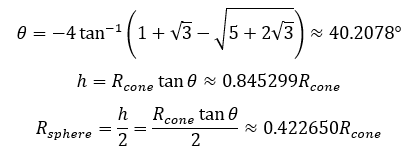Solution

1.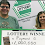2.